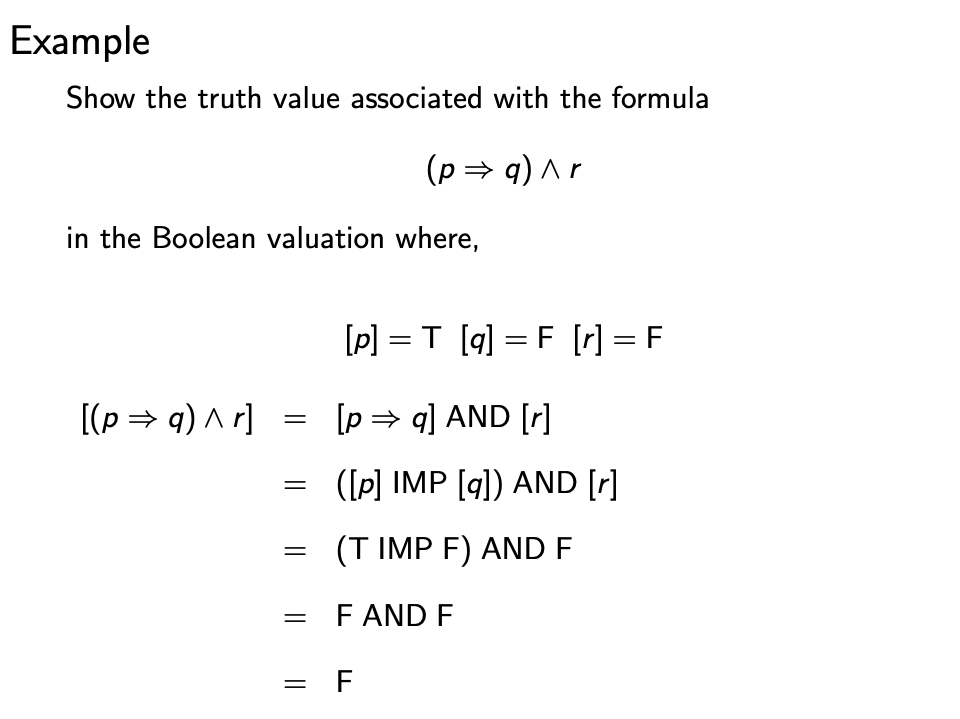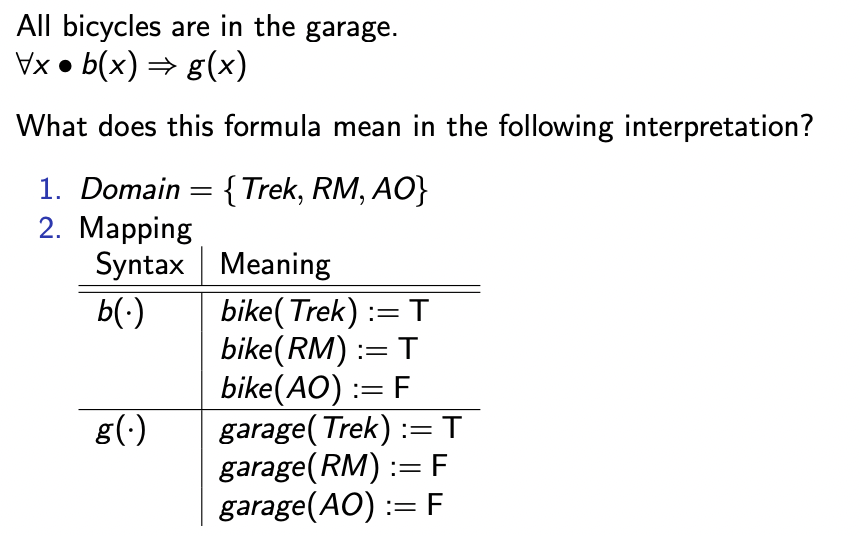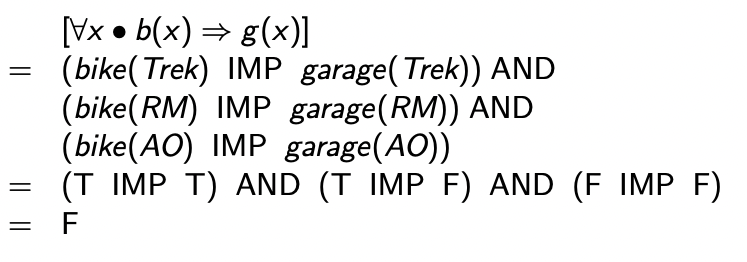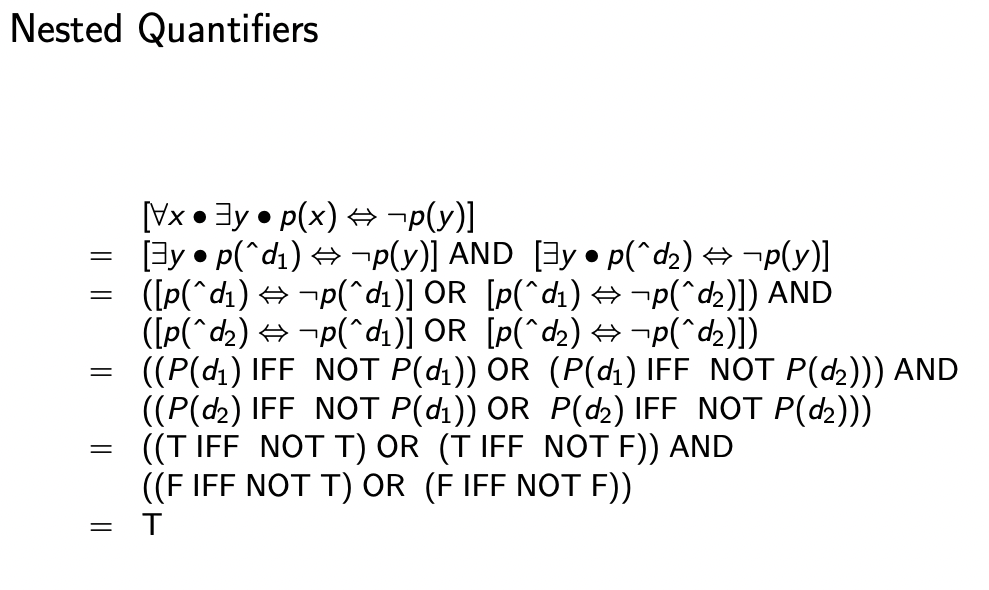Logic

# Semantics §

This is a very confusing topic to wrap my head around, because I confuse them with the idea of syntax.

I think I finally get it!!

Analogy with English Sentence

Take any english sentence: “The lake is blue”. Think of syntax as the arrangement of spaces and characters which form up the words of the sentence.

If you didn’t speak english (semantics didn’t exist), then the syntax would seem like gibberish to you. It’s only because you have Semantics, i.e. you gave meaning to certain arrangements of characters, that you can understand and analyze an English sentence.

• “blue” represents this abstract concept of the color blue
• “lake” represents this idea of the a group of water

So it’s really interesting to ponder at, how can we teach Artificial Intelligence semantics?

Semantics means “meaning”. They define “truth” for a logic.

Semantics provide an interpretation (mapping) of values in one world to values in another world. Semantics are often a function from expressions in one world to expressions in another world.

The semantics of a Logic defines the meaning of formulas in the logic leading to a definition of the symbol: “” (pronounced “entails” or “valid” or “semantic entailment”).

means that from the premises we may conclude , where and are all wff

This is NOT to be confused with Proof Theory, which is interested in constructing proofs.

Semantics are often a function from expressions in one world to expressions in another world.

The two worlds we are relating here are

1. the wff of the syntax and
2. the set of truth values and functions on these truth values

is a meta-symbol, meaning not a symbol that can be used in a formula of the logic but a symbol about formulas in the logic.

I struggled with this over the midterm.

### Interpreting Semantics of Propositional Logic / Showing the Truth Value §

Semantics are needed to show the truth value of something. Remember, the Boolean Valuation gives us the actual values.### Interpreting Semantics of Untyped Predicate Logic §

For Untyped Predicate Logic, things are a bit more complicated then just using a truth table.

We can interpret a logic formula if we know the values. Ahh, this is what they ask you to do when they say: “Provide an interpretation to show to this argument is invalid”

• So basically showing a counterexample

We describe the meaning of a predicate logic formula in a particular interpretation. An interpretation includes the following:

1. Mapping (for every constant, function, predicate) | Syntax | Meaning | | :---: | :---: | | Constants | each maps to a value of the domain | | Functions | each maps to a total function of matching arity that takes arguments from the domain, and returns values in the domain | | Predicates | each maps to a total predicate of matching arity that takes arguments from the domain, and returns values of Tr |

For example,You see how on the left, we use lowercase? Because it’s syntax! On the right, because that is actual meaning, i.e. the Semantics, then we use a different notation.

Quantifiers:

• When you have universally quantified , you AND all the possible values of the domain
• When you have existentially quantified variable , you OR all the terms

For example,Example in George:

(exists x . g(x) & b(k(x))) & !b(c)

An interpretation in which this formula is T:

1) Domain = {d1 , d2}

2) Syntax | Meaning
−−−−−−−−−−−−−−−−−−
c   | d1
b (.)   | B( d1 ) := F
| B( d2 ) := T
g(.)    | G( d1 ) := T
| G( d2 ) := T
k (.)   | K( d1 ) := d1
| K( d2 ) := d2

Demonstration:
[(exists  x . g(x)  & b(k(x))) & !b(c)]
= [exists x . g(x) & b(k(x))] AND NOT [b(c)]
= ([g(ˆd1) & b(k(ˆd1))] OR [g(ˆd2) & b(k(ˆd2))]) AND NOT [b(c)] = ((G(d1) AND B(K(d1))) OR (G(d2) AND B(K(d2)))) AND NOT B(d1)
= ((G(d1) AND B(d1)) OR (G(d2) AND B(d2)) AND NOT B(d1)
= ((T AND F) OR (T AND T)) AND NOT F
= T
• Notice the ^, the ˆd notation allows us to do the substitution in place, but still respect the distinction between the syntax and the semantics.
• However, I think we can just go for the jump from syntax to semantics

Another example, make sure you really understand this because it’s important for final exam.